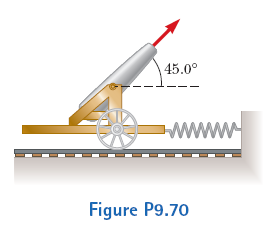# Problem: A cannon is rigidly attached to a carriage, which can move along horizontal rails but is connected to a post by a large spring, initially unstretched and with force constant k = 2.00 X 104 N/m, as shown in the figure. The cannon fires a 200-kg projectile at a velocity of 125 m/s directed 45.0° above the horizontal. Assuming that the mass of the cannon and its carriage is 5000 kg,(a) Find the recoil speed of the cannon.(b) Determine the maximum extension of the spring.(c) Find the maximum force the spring exerts on the carriage.(d) Consider the system consisting of the cannon, carriage, and projectile. Is the momentum of this system conserved during the firing? Why or why not?

###### FREE Expert Solution
86% (437 ratings)
###### Problem Details

A cannon is rigidly attached to a carriage, which can move along horizontal rails but is connected to a post by a large spring, initially unstretched and with force constant k = 2.00 X 104 N/m, as shown in the figure. The cannon fires a 200-kg projectile at a velocity of 125 m/s directed 45.0° above the horizontal. Assuming that the mass of the cannon and its carriage is 5000 kg,
(a) Find the recoil speed of the cannon.
(b) Determine the maximum extension of the spring.
(c) Find the maximum force the spring exerts on the carriage.
(d) Consider the system consisting of the cannon, carriage, and projectile. Is the momentum of this system conserved during the firing? Why or why not?Frequently Asked Questions

What scientific concept do you need to know in order to solve this problem?

Our tutors have indicated that to solve this problem you will need to apply the Push-Away Problems With Energy concept. You can view video lessons to learn Push-Away Problems With Energy. Or if you need more Push-Away Problems With Energy practice, you can also practice Push-Away Problems With Energy practice problems.

How long does this problem take to solve?

Our expert Physics tutor, Julia took 4 minutes and 22 seconds to solve this problem. You can follow their steps in the video explanation above.

What professor is this problem relevant for?

Based on our data, we think this problem is relevant for Professor Maillis' class at UWM.

What textbook is this problem found in?

Our data indicates that this problem or a close variation was asked in Physics for Scientists and Engineers - Serway Calc 9th Edition. You can also practice Physics for Scientists and Engineers - Serway Calc 9th Edition practice problems.